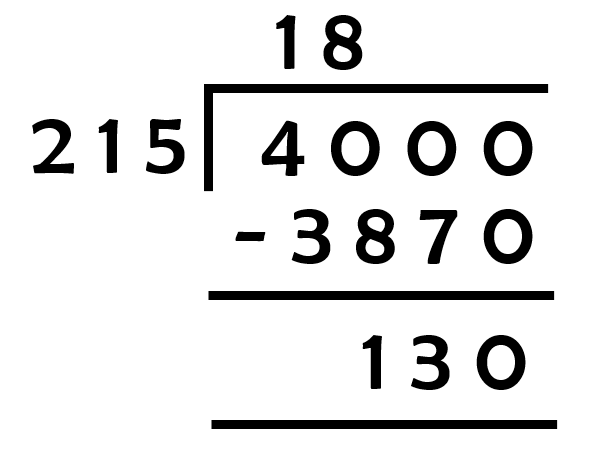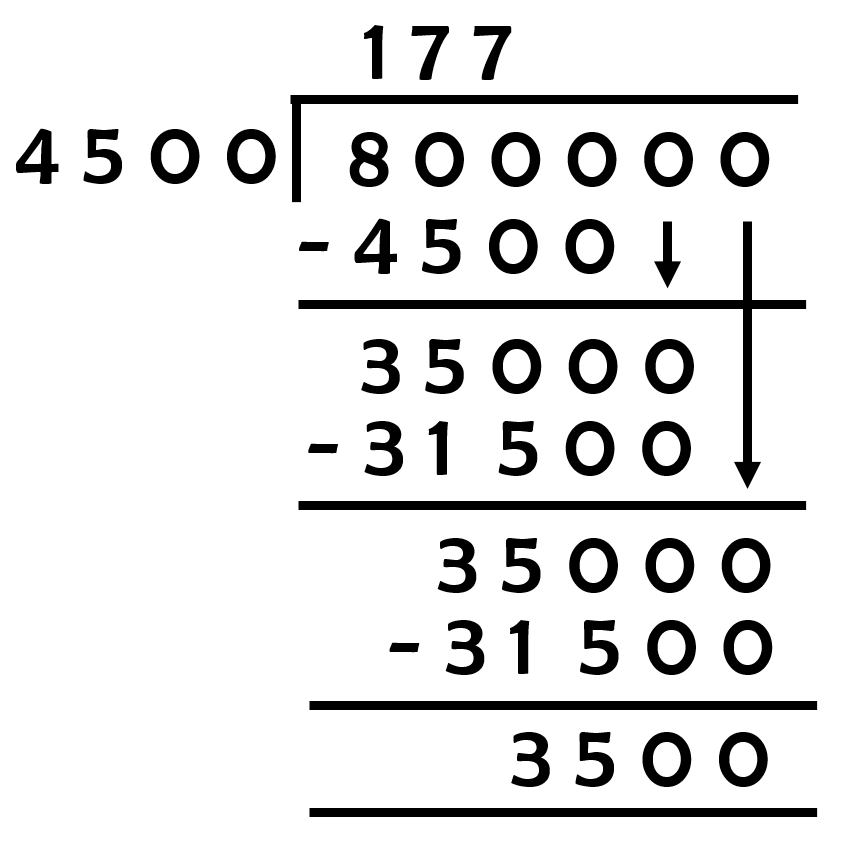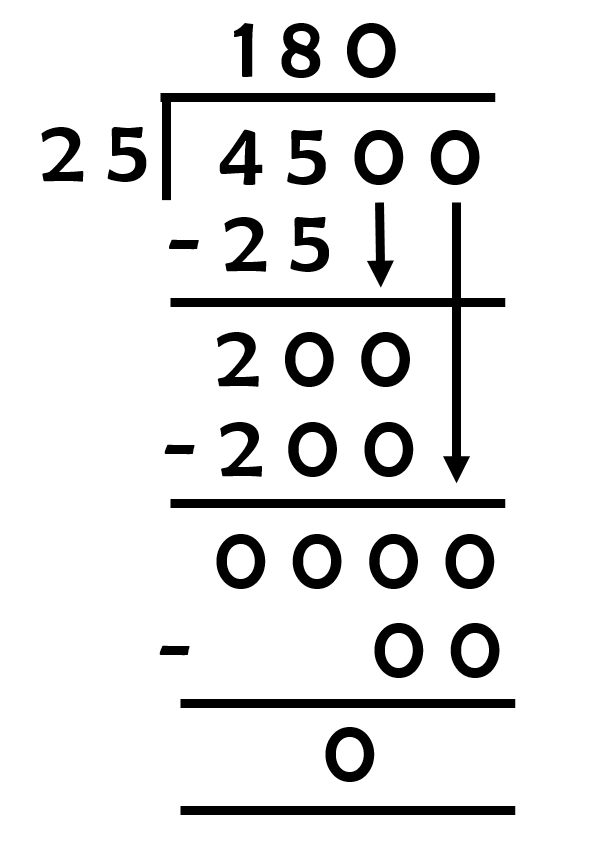# vs.eyeandcontacts.com

## Chapter 1 Knowing our Numbers Exercise 1.2

Question 1: A book exhibition was held for four days in a school. The number of tickets sold at the counter on the first, second, third and final day was respectively 1094, 1812, 2050 and 2751. Find the total number of tickets sold on all the four days.

Number of tickets sold on first day = 1094
Number of tickets sold on second day = 1812
Number of tickets sold on third day = 2050
Number of tickets sold on final day = 2751
Number of tickets sold on all four days = ?

= 1094 + 1812 + 2050 + 2751 = 7707
Therefore, the total number of tickets sold on all the four days is 7707.

Question 2: Shekhar is a famous cricket player. He has so far scored 6980 runs in test matches. He wishes to complete 10,000 runs. How many more runs does he need?

Total runs scored by Shekhar = 6980
Total runs to be scored by Shekhar = 10000
Runs needed to complete the target = ?

= 10000 - 6980 = 3020
Therefore, the runs need to be completed by Shekhar is 3020.

Question 3: In an election, the successful candidate registered 5,77,500 votes and his nearest rival secured 3,48,700 votes. By what margin did the successful candidate win the election?

Number of votes the successful candidate registered = 577500
Number of votes the successful candidate’s rival registered = 348700
Margin by which he won the election = ?

= 577500 - 348700 = 228800
Therefore, the margin by which the successful candidate won against his rival is 228800.

Question 4: Kirti bookstore sold books worth ₹2,85,891 in the first week of June and books worth ₹4,00,768 in the second week of the month. How much was the sale for the two weeks together? In which week was the sale greater and by how much?

Cost of books sold by Kirti bookstore in the first week of June = ₹285891
Cost of books sold by Kirti bookstore in the second week of June = ₹400768
Total cost of books sold by Kirti bookstore in first 2 weeks off June = ?

= ₹400768 + ₹285891
= ₹686659
Therefore, the sale for the two week together was ₹686659.

Comparing
= ₹400768 > ₹285891
Therefore, the sale was more in the 2nd week.

= ₹400768 - ₹285891
= ₹114877
Therefore, the difference between the 2 weeks was ₹114877.

Question 5: Find the difference between the greatest and the least 5-digit number that can be written using the digits 6, 2, 7, 4, 3 each only once.

Greatest number made with digits 6, 2, 7, 4, 3 = 76432
Smallest number made with digits 6, 2, 7, 4, 3 = 23467
Difference between those 2 numbers = ?

= 76432 - 23467 = 52965
Therefore, the difference between the greatest and the smallest 5 digit number made with 6, 2, 7, 4, 3 is 52965.

Question 6: A machine, on an average, manufactures 2,825 screws a day. How many screws did it produce in the month of January 2006?

Number of screws produced by machine in a day = 2825
Number of screws produced by machine in month of January 2006 = ?
Number of days in January = 31

= 2825 × 31
= 87575
Therefore, the total number of screws produced by the machine in the month of January 2006 is 87575.

Question 7: A merchant had ₹78,592 with her. She placed an order for purchasing 40 radio sets at ₹1200 each. How much money will remain with her after the purchase?

Money with merchant = ₹78592
Number of radios purchased by her = 40
Cost of 1 radio = ₹1200
Money left with merchant = ?

= 1200 × 40
= 48000
Therefore, the cost of 40 radios is ₹48000.

Money left with her = Total money she had in beginning - Total money spent on radios
= ₹78592 - ₹48000 = ₹30592

Therefore, the total money left with merchant is ₹30592.

Question 8: A student multiplied 7236 by 65 instead of multiplying by 56. By how much was his answer greater than the correct answer? (Hint: Do you need to do both the multiplications?)

Student multiplication
= 7236 × 65
= 470340

Actual multiplication
= 7236 × 56
= 405,216

Difference between Student’s multiplication and actual multiplication = ?
= 470340 - 405216 = 65,124

Question 9: To stitch a shirt, 2 m 15 cm cloth is needed. Out of 40 m cloth, how many shirts can be stitched and how much cloth will remain? (Hint: convert data in cm.)

Length of cloth required to stitch a shirt = 2 m 15 cm
Total length of cloth = 40 m

Conversion
1 m = 100 cm
= 2 m 15 cm = 2.15 × 100 = 215 cm
= 40 m = 40 × 100 = 4000 cm
= 4000/215= 130 cm is left
= 130/100 = 1.3 m
Therefore, 18 shirts can be stitched and 1.3 m of cloth will be left out.

Question 10: Medicine is packed in boxes, each weighing 4 kg 500g. How many such boxes can be loaded in a van which cannot carry beyond 800 kg?

Weight of medicine = 4 kg 500 g
Capacity of van to carry = 800 kg

Conversion
1 kg = 1000 g
= 4 kg 500 g = 4.5 × 1000 = 4500 g
= 800 kg = 800 × 1000 = 800000
= 800000/4500= 3500 g is left
= 3500/1000 = 3.5 kg
Therefore, 177 boxes can be loaded into van and 3.5 kg of capacity of van will be left out.

Question 11: The distance between the school and a student’s house is 1 km 875 m. Everyday she walks both ways. Find the total distance covered by her in six days.

Distance between student’s house and school = 1 km 875 m (1875 m)
Distance walked every day = 2 × 1875 = 3750 m
Total distance covered by her in 6 days = ?

= 3750 × 6 = 22500 m
= 22500/1000 = 22.5 km
Therefore, the distance covered by the student in 6 days is 22.5 km or 22500 m.

Question 12: A vessel has 4 litres and 500 ml of curd. In how many glasses, each of 25 ml capacity, can it be filled?

Curd in a vessel = 4 lt 500 ml
Capacity of glass = 25 ml
Number of glasses required = ?

Conversion
1 lt = 1000 g
= 4 lt 500 ml = 4.5 × 1000 = 4500 ml
= 4500/25= 180 glasses are required
Therefore, 180 glasses each of 25 ml capacity can the curd it be filled.